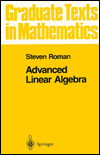#Advanced Linear Algebra, Third Edition 2008

## Springer-Verlag Graduate Texts in Mathematics, Volume 135

### Preface to Third Edition

Let me begin by thanking the readers of the second edition for their many helpful comments and suggestions, with special thanks to Joe Kidd and Nam Trang. For the third edition, I have corrected all known errors, polished and refined some arguments (such as the discussion of reflexivity, the rational canonical form, best approximations and the definitions of tensor products) and upgraded some proofs that were originally done only for finite-dimensional/rank cases. I have also moved some of the material on projection operators to an earlier position in the text.

A few new theorems have been added in this edition, including the spectral mapping theorem and a theorem to the effect that the dimension of a vector space V is less than or equal to the dimension of the dual space V*, with equality if and only if V is finite-dimensional.

I have also added a new chapter on associative algebras that includes the well-known characterizations of the finite-dimensional division algebras over the real field (a theorem of Frobenius) and over a finite field (Wedderburn's theorem). The reference section has been enlarged considerably, with over a hundred references to books on linear algebra.

### Preface to Second Edition

The text has been completely rewritten. I hope that an additional 12 years and roughly 20 books worth of experience has enabled me to improve the quality of my exposition. Also, the exercise sets have been completely rewritten.

The second edition contains two new chapters: a chapter on convexity, separation and positive solutions to linear systems (Chapter 15) and a chapter on the QR decomposition, singular values and pseudoinverses (Chapter 17). The treatments of tensor products and the umbral calculus have been greatly expanded and I have included discussions of determinants (in the chapter on tensor products), the complexification of a real vector space, Schur's lemma and Geršgorin disks.

### Preface to First Edition

This is a graduate level textbook covering an especially broad range of topics. The first part of the book contains a careful but rapid discussion of the basics of linear algebra, including vector spaces, linear transformations, quotient spaces, and isomorphism theorems. The author then proceeds to a discussion of modules, emphasizing a comparison with vector spaces. A thorough discussion of inner product spaces, eigenvalues, eigenvectors, and finite dimensional spectral theory follows, culminating in the finite dimensional spectral theorem for normal operators.

The second part of the book is a collection of topics, including metric vector spaces, metric spaces, Hilbert spaces, tensor products, and affine geometry. The final chapter contains a discussion of the umbral calculus, a relatively new area of modern algebra that is important in applications. This is the first time that this topic has appeared in a textbook. The book contains numerous exercises, and is suitable both as a textbook and as a reference for students and instructors.